# Solving Systems by Elimination Module 6 3 Objectives

• Slides: 22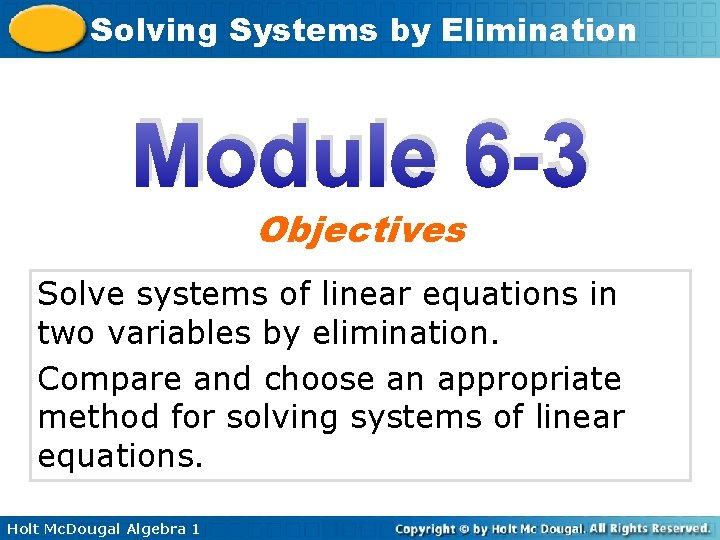Solving Systems by Elimination Module 6 -3 Objectives Solve systems of linear equations in two variables by elimination. Compare and choose an appropriate method for solving systems of linear equations. Holt Mc. Dougal Algebra 1Solving Systems by Elimination Another method for solving systems of equations is elimination. Like substitution, the goal of elimination is to get one equation that has only one variable. When you use the elimination method to solve a system of linear equations, align all like terms in the equations. Then determine whether any like terms can be eliminated because they have opposite coefficients. Holt Mc. Dougal Algebra 1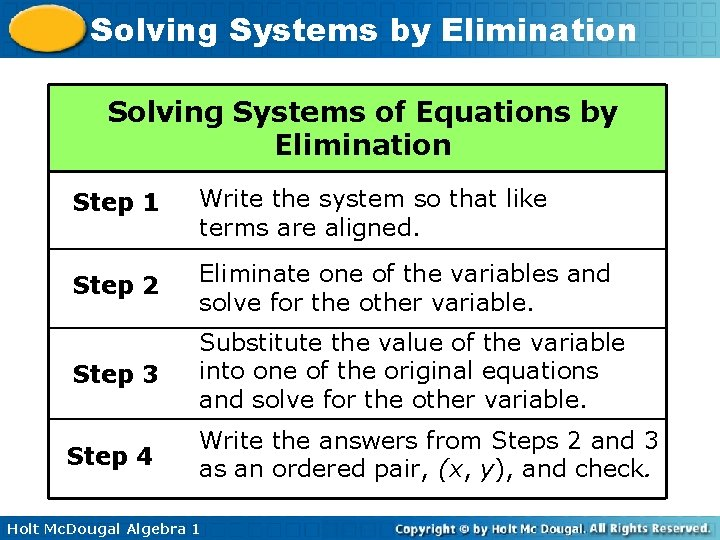Solving Systems by Elimination Solving Systems of Equations by Elimination Step 1 Write the system so that like terms are aligned. Step 2 Eliminate one of the variables and solve for the other variable. Step 3 Substitute the value of the variable into one of the original equations and solve for the other variable. Step 4 Write the answers from Steps 2 and 3 as an ordered pair, (x, y), and check. Holt Mc. Dougal Algebra 1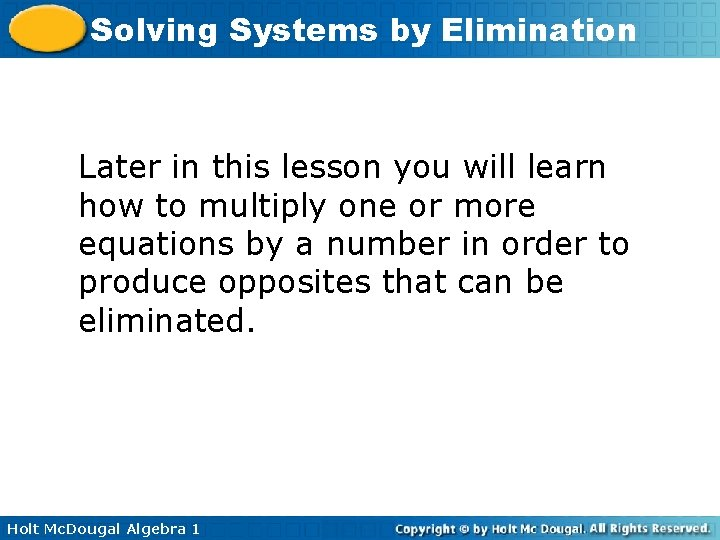Solving Systems by Elimination Later in this lesson you will learn how to multiply one or more equations by a number in order to produce opposites that can be eliminated. Holt Mc. Dougal Algebra 1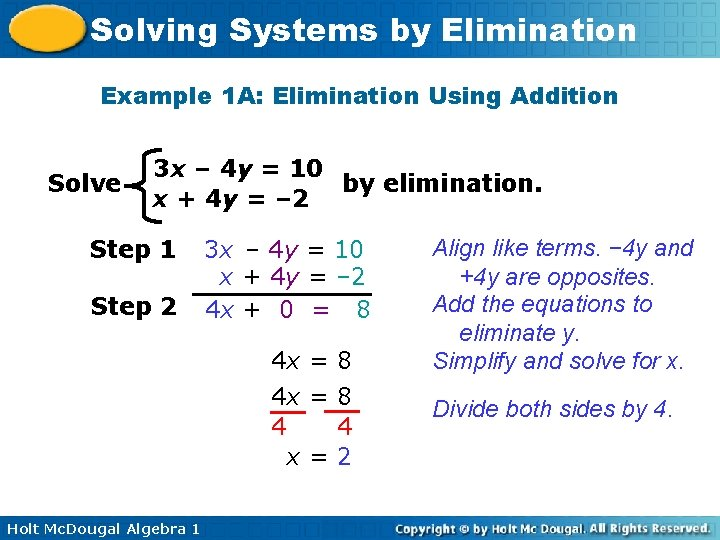Solving Systems by Elimination Example 1 A: Elimination Using Addition Solve 3 x – 4 y = 10 by elimination. x + 4 y = – 2 Step 1 Step 2 3 x – 4 y = 10 x + 4 y = – 2 4 x + 0 = 8 4 x = 8 4 4 x=2 Holt Mc. Dougal Algebra 1 Align like terms. − 4 y and +4 y are opposites. Add the equations to eliminate y. Simplify and solve for x. Divide both sides by 4.Solving Systems by Elimination Example 1 A Continued Step 3 x + 4 y = – 2 2 + 4 y = – 2 – 2 4 y = – 4 4 y – 4 4 4 y = – 1 Step 4 (2, – 1) Holt Mc. Dougal Algebra 1 Write one of the original equations. Substitute 2 for x. Subtract 2 from both sides. Divide both sides by 4. Write the solution as an ordered pair.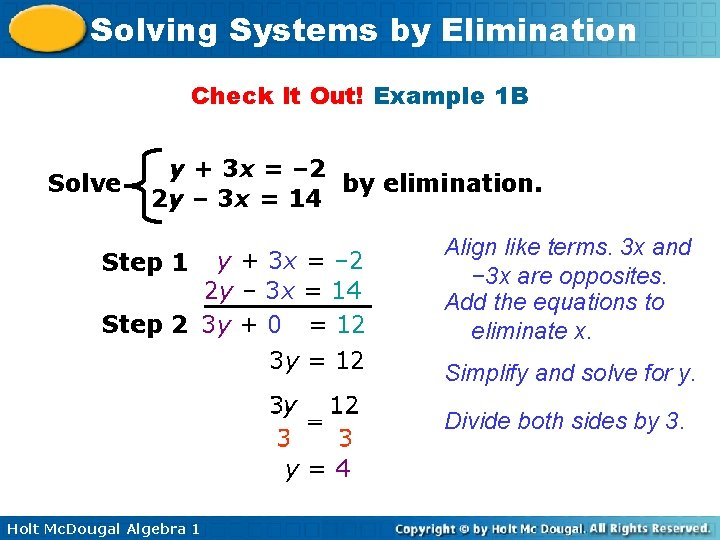Solving Systems by Elimination Check It Out! Example 1 B Solve y + 3 x = – 2 by elimination. 2 y – 3 x = 14 y + 3 x = – 2 2 y – 3 x = 14 Step 2 3 y + 0 = 12 3 y = 12 Step 1 Align like terms. 3 x and − 3 x are opposites. Add the equations to eliminate x. Simplify and solve for y. Divide both sides by 3. y=4 Holt Mc. Dougal Algebra 1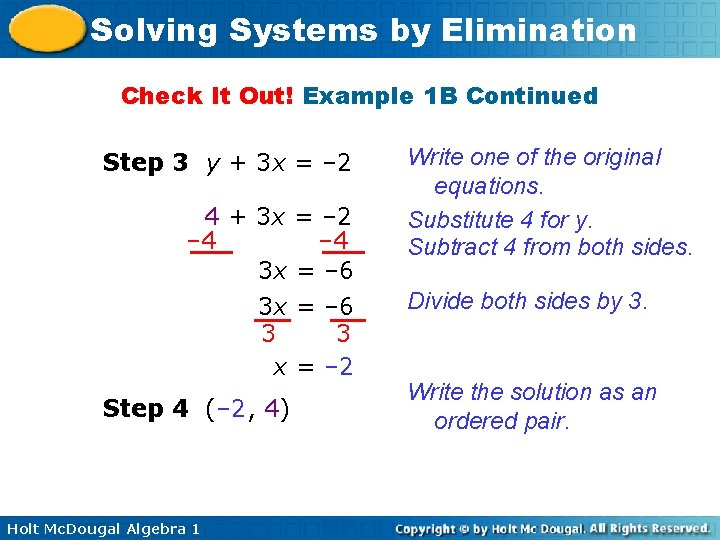Solving Systems by Elimination Check It Out! Example 1 B Continued Step 3 y + 3 x = – 2 4 + 3 x = – 2 – 4 3 x = – 6 3 3 x = – 2 Step 4 (– 2, 4) Holt Mc. Dougal Algebra 1 Write one of the original equations. Substitute 4 for y. Subtract 4 from both sides. Divide both sides by 3. Write the solution as an ordered pair.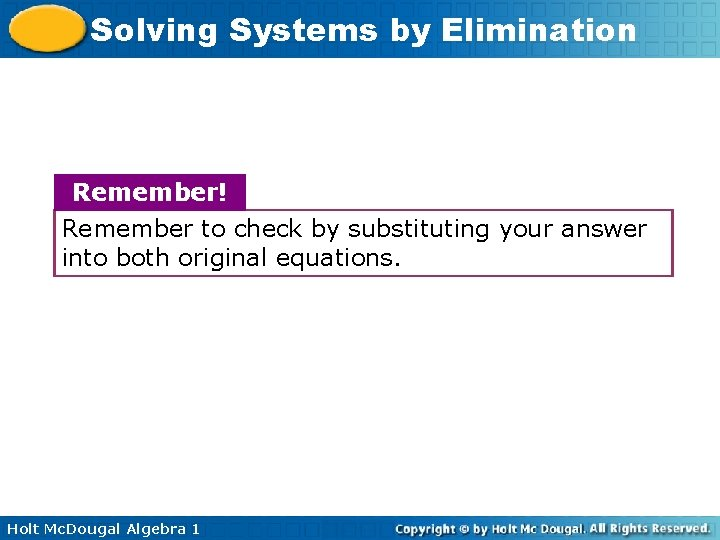Solving Systems by Elimination Remember! Remember to check by substituting your answer into both original equations. Holt Mc. Dougal Algebra 1Solving Systems by Elimination In some cases, you will first need to multiply one or both of the equations by a number so that one variable has opposite coefficients. Holt Mc. Dougal Algebra 1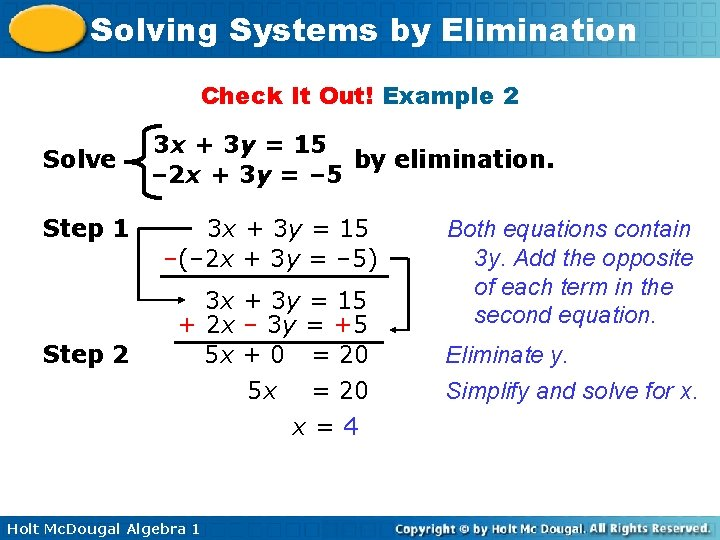Solving Systems by Elimination Check It Out! Example 2 Solve Step 1 Step 2 3 x + 3 y = 15 by elimination. – 2 x + 3 y = – 5 3 x + 3 y = 15 –(– 2 x + 3 y = – 5) 3 x + 3 y = 15 + 2 x – 3 y = +5 5 x + 0 = 20 5 x = 20 x=4 Holt Mc. Dougal Algebra 1 Both equations contain 3 y. Add the opposite of each term in the second equation. Eliminate y. Simplify and solve for x.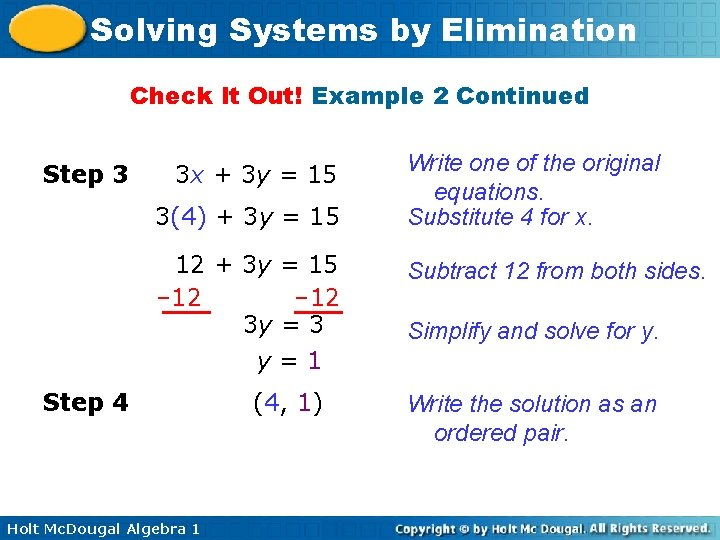Solving Systems by Elimination Check It Out! Example 2 Continued Step 3 3 x + 3 y = 15 3(4) + 3 y = 15 12 + 3 y = 15 – 12 3 y = 3 y=1 Step 4 Holt Mc. Dougal Algebra 1 (4, 1) Write one of the original equations. Substitute 4 for x. Subtract 12 from both sides. Simplify and solve for y. Write the solution as an ordered pair.Solving Systems by Elimination Example 3 A: Elimination Using Multiplication First Solve the system by elimination. x + 2 y = 11 – 3 x + y = – 5 Step 1 Step 2 x + 2 y = 11 – 2(– 3 x + y = – 5) x + 2 y = 11 +(6 x – 2 y = +10) 7 x + 0 = 21 7 x = 21 x=3 Holt Mc. Dougal Algebra 1 Multiply each term in the second equation by – 2 to get opposite y-coefficients. Add the new equation to the first equation to eliminate y. Solve for x.Solving Systems by Elimination Example 3 A Continued Step 3 x + 2 y = 11 3 + 2 y = 11 – 3 2 y = 8 y=4 Step 4 Holt Mc. Dougal Algebra 1 (3, 4) Write one of the original equations. Substitute 3 for x. Subtract 3 from both sides. Solve for y. Write the solution as an ordered pair.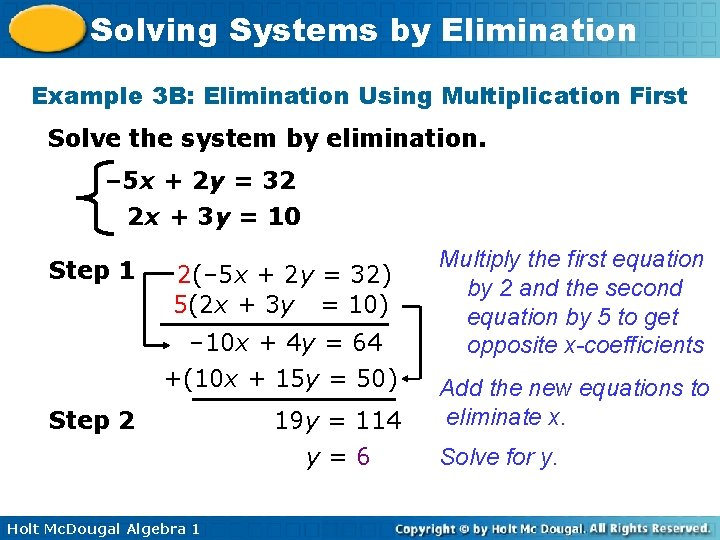Solving Systems by Elimination Example 3 B: Elimination Using Multiplication First Solve the system by elimination. – 5 x + 2 y = 32 2 x + 3 y = 10 Step 1 2(– 5 x + 2 y = 32) 5(2 x + 3 y = 10) – 10 x + 4 y = 64 +(10 x + 15 y = 50) Step 2 Holt Mc. Dougal Algebra 1 19 y = 114 y=6 Multiply the first equation by 2 and the second equation by 5 to get opposite x-coefficients Add the new equations to eliminate x. Solve for y.Solving Systems by Elimination Example 3 B Continued Step 3 2 x + 3 y = 10 2 x + 3(6) = 10 2 x + 18 = 10 – 18 Step 4 Holt Mc. Dougal Algebra 1 2 x = – 8 x = – 4 (– 4, 6) Write one of the original equations. Substitute 6 for y. Subtract 18 from both sides. Solve for x. Write the solution as an ordered pair.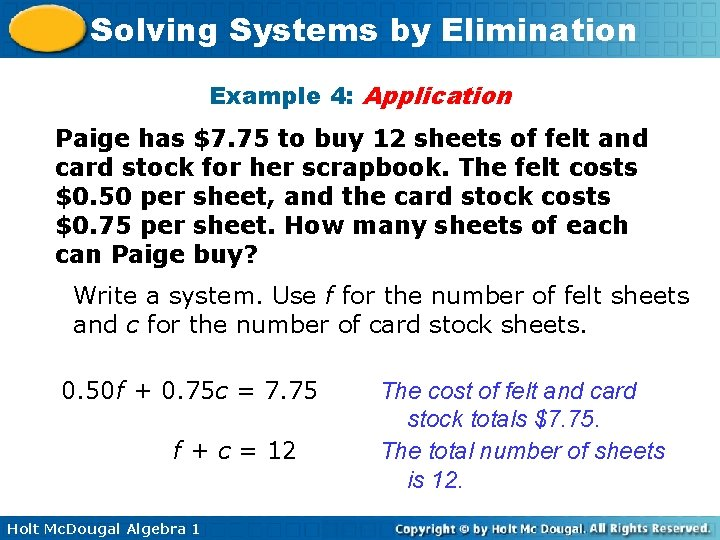Solving Systems by Elimination Example 4: Application Paige has \$7. 75 to buy 12 sheets of felt and card stock for her scrapbook. The felt costs \$0. 50 per sheet, and the card stock costs \$0. 75 per sheet. How many sheets of each can Paige buy? Write a system. Use f for the number of felt sheets and c for the number of card stock sheets. 0. 50 f + 0. 75 c = 7. 75 f + c = 12 Holt Mc. Dougal Algebra 1 The cost of felt and card stock totals \$7. 75. The total number of sheets is 12.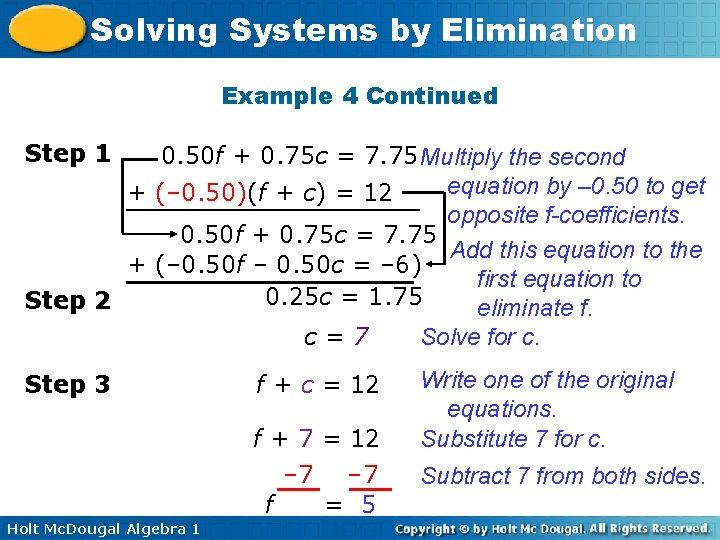Solving Systems by Elimination Example 4 Continued Step 1 0. 50 f + 0. 75 c = 7. 75 Multiply the second equation by – 0. 50 to get + (– 0. 50)(f + c) = 12 opposite f-coefficients. 0. 50 f + 0. 75 c = 7. 75 Add this equation to the + (– 0. 50 f – 0. 50 c = – 6) first equation to 0. 25 c = 1. 75 Step 2 eliminate f. Solve for c. c=7 Step 3 f + c = 12 f + 7 = 12 – 7 f = 5 Holt Mc. Dougal Algebra 1 Write one of the original equations. Substitute 7 for c. Subtract 7 from both sides.Solving Systems by Elimination Example 4 Continued (7, 5) Write the solution as an ordered pair. Paige can buy 7 sheets of card stock and 5 sheets of felt. Step 4 Holt Mc. Dougal Algebra 1Solving Systems by Elimination All systems can be solved in more than one way. For some systems, some methods may be better than others. Holt Mc. Dougal Algebra 1Solving Systems by Elimination Holt Mc. Dougal Algebra 1Solving Systems by Elimination Tonight’s HW: p. 151 #1 -19 odds, p. 152 #25, 27 Holt Mc. Dougal Algebra 1